# NCERT Solutions for Class 10 Maths:Chapter 6 Similar triangles EXERCISE 6.2

In this page we have NCERT Solutions for Class 10 Mathematics:Chapter 6 Similar triangles for EXERCISE 6.2 on pages 128 and 129. Hope you like them and do not forget to like , social_share and comment at the end of the page.
Note: All the questions are based Basic Proportionality Theorem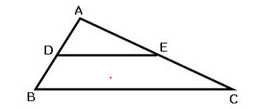If a line is drawn parallel to one side of a triangle intersecting the other two sides, then it divides the two sides in the same ratio.

In the $\Delta ABC$ , if DE || BC
i. $\frac {AD}{DB}= \frac {AE}{EC}$
ii. $\frac {AD}{AB}= \frac {AE}{AC}$
iii. $\frac {DB}{AB}= \frac {EC}{AC}$

## Triangles EXERCISE 6.2

Question 1.
In below figure (i) and (ii), DE || BC. Find EC in (i) and AD in (ii).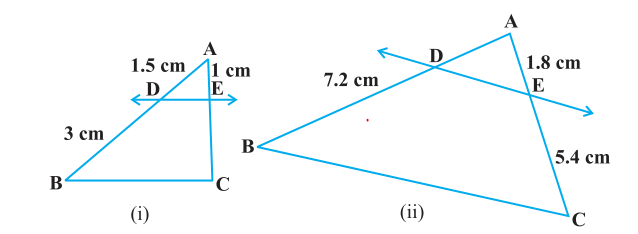Solution
(i) In ΔABC, DE||BC
Now from Basic proportionality theorem
$\frac {AD}{DB} = \frac {AE}{EC}$
$\frac {1.5}{3} = \frac {1}{EC}$
EC = 3/1.5
EC = 2 = 2 cm
(ii) In ΔABC, DE||BC
Now from Basic proportionality theorem
$\frac {AD}{DB} = \frac {AE}{EC}$
$\frac {AD}{7.2} = \frac {1.8}{5.4}$
AD = 1.8×7.2/5.4 = 2.4 cm

Question 2
E and F are points on the sides PQ and PR respectively of a ΔPQR. For each of the following cases, state whether EF || QR.
(i) PE = 3.9 cm, EQ = 3 cm, PF = 3.6 cm and FR = 2.4 cm
(ii) PE = 4 cm, QE = 4.5 cm, PF = 8 cm and RF = 9 cm
(iii) PQ = 1.28 cm, PR = 2.56 cm, PE = 0.18 cm and PF = 0.63 cm
Solution
In ΔPQR, E and F are two points on side PQ and PR respectively.
(i) Given
PE = 3.9 cm, EQ = 3 cm, PF = 3.6 cm, FR = 2,4 cm
Now the Ratio PE/EQ = 3.9/3 = 39/30 = 13/10 = 1.3
And, PF/FR = 3.6/2.4 = 36/24 = 3/2 = 1.5
So, $\frac {PE}{EQ} \neq \frac {PF}{FR}$
So, as per converse of Basic proportionality theorem
Hence, EF is not parallel to QR.
(ii) Given
PE = 4 cm, QE = 4.5 cm, PF = 8cm, RF = 9cm
Now the ratio PE/QE = 4/4.5 = 40/45 = 8/9
And PF/RF = 8/9
So, $\frac {PE}{EQ} = \frac {PF}{FR}$
So as per converse of Basic proportionality theorem
Hence, EF is parallel to QR.
(iii) Given PQ = 1.28 cm, PR = 2.56 cm, PE = 0.18 cm, PF = 0.36 cm
Here, EQ = PQ - PE = 1.28 - 0.18 = 1.10 cm
And, FR = PR - PF = 2.56 - 0.36 = 2.20 cm
So, PE/EQ = 0.18/1.10 = 18/110 = 9/55
And, PE/FR = 0.36/2.20 = 36/220 = 9/55
so, $\frac {PE}{EQ} = \frac {PF}{FR}$
So as per converse of Basic proportionality theorem
Hence, EF is parallel to QR.
Question 3
In the below figure, if LM || CB and LN || CD, prove that AM/MB = AN/AD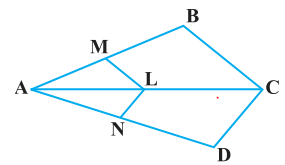Solution
In the given figure, LM || CB
By using basic proportionality theorem, we get,
AM/MB = AL/LC ... (i)
Similarly, LN || CD
By using basic proportionality theorem, we get,
From (i) and (ii), we get
Question 4
In the below figure DE||AC and DF||AE. Prove that BF/FE = BE/EC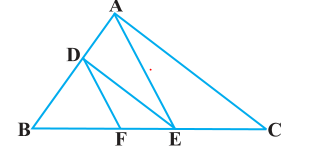Solution
In ΔABC, DE || AC
By using Basic Proportionality Theorem
BD/DA = BE/EC ...(i)
In   ΔABC, DF || AE
By using Basic Proportionality Theorem
BD/DA = BF/FE ...(ii)
From equation (i) and (ii), we get
BE/EC = BF/FE

Question 5
In the below figure, DE||OQ and DF||OR, show that EF||QR.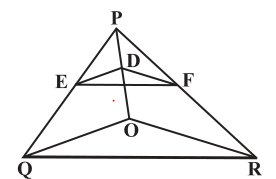Solution
In ΔPQO, DE || OQ
By using Basic Proportionality Theorem
$\frac {PD}{DO} = \frac {PE}{EQ}$ ...(i)
In ΔPRO, DF||OR
By using Basic Proportionality Theorem
$\frac {PD}{DO} = \frac {PF}{FR}$  ...(ii)
From equation (i) and (ii), we get
$\frac {PE}{EQ} = \frac {PF}{FR}$
By converse of Basic Proportionality Theorem
So In ΔPQR, EF || QR.

Question 6
In the below figure, A, B and C are points on OP, OQ and OR respectively such that AB || PQ and AC || PR. Show that BC || QR.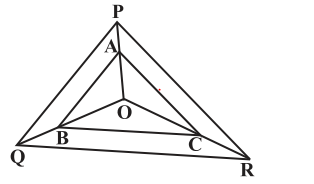Solution
In ΔOPQ, AB || PQ
By using Basic Proportionality Theorem
$\frac {OA}{AP}= \frac {OB}{BQ}$ ...(i)
In ΔOPR, AC || PR
By using Basic Proportionality Theorem
$\frac {OA}{AP}= \frac {OC}{CR}...(ii) From equation (i) and (ii), we get$ \frac {OB}{BQ} = \frac {OC}{CR}$By converse of Basic Proportionality Theorem So In ΔOQR, BC || QR. Question 7 Using Basic proportionality theorem, prove that a line drawn through the mid-points of one side of a triangle parallel to another side bisects the third side. (Recall that you have proved it in Class IX). Solution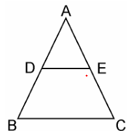ΔABC in which D is the mid-point of AB such that AD=DB. A line parallel to BC intersects AC at E as shown in above figure such that DE || BC. To Prove: E is the mid-point of AC. Proof: D is the mid-point of AB. ∴ AD=DB ⇒ AD/BD = 1 ... (i) In ΔABC, DE || BC, By using Basic Proportionality Theorem AD/DB = AE/EC Now as AD/BD=1 AE/EC=1 So E is the mid-point of AC Question 8 Using Converse of basic proportionality theorem, prove that the line joining the mid-points of any two sides of a triangle is parallel to the third side. (Recall that you have done it in Class IX). SolutionΔABC in which D and E are the mid points of AB and AC respectively such that AD=BD and AE=EC. To Prove: DE || BC Proof: D is the midpoint of AB (Given) So AD=DB Then AD/BD = 1 ... (i) Also, E is the mid-point of AC (Given) So AE=EC Then AE/EC = 1 [From equation (i)] From equation (i) and (ii), we get AD/BD = AE/EC By converse of Basic Proportionality Theorem Hence, DE || BC Question 9. ABCD is a trapezium in which AB || DC and its diagonals intersect each other at the point O. Prove that$ \frac {AO}{BO} = \frac {CO}{DO}$Solution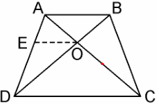Given: ABCD is a trapezium in which AB || DC in which diagonals AC and BD intersect each other at O. Through O, draw EO || DC Now as DC || AB, So, EO||AB In ΔADC, we have OE || DC (By Construction) By using Basic Proportionality Theorem$ \frac {AE}{ED} = \frac {AO}{CO}$...(i) In ΔABD, we have OE || AB (Proved above) By using Basic Proportionality Theorem$ \frac {DE}{EA} = \frac {DO}{BO}$...(ii) From equation (i) and (ii), we get$\frac {AO}{CO} = \frac {BO}{DO} \frac {AO}{BO} = \frac {CO}{DO}$Question 10 The diagonals of a quadrilateral ABCD intersect each other at the point O such that$ \frac {AO}{BO} = \frac {CO}{DO}$. Show that ABCD is a trapezium. SolutionAccording to the question Quadrilateral ABCD in which diagonals AC and BD intersects each other at O such that$ \frac {AO}{BO} = \frac {CO}{DO}$--- -(i) Through O, draw line EO, where EO || AB, which meets AD at E. In ΔDAB, we have EO || AB [By using Basic Proportionality Theorem$ \frac {DE}{EA} = \frac {DO}{OB}$...(ii) From (i)$ \frac {AO}{CO} = \frac {BO}{DO}$Rearranging the equation,we get$ \frac {CO}{AO} = \frac {BO}{DO} \frac {DO}{OB} = \frac {CO}{AO}$...(iii) From equation (ii) and (iii), we get$ \frac {DE}{EA} = \frac {CO}{AO}$By using converse of Basic Proportionality Theorem Therefore, EO || DC Now EO || AB So AB || DC. Hence, quadrilateral ABCD is a trapezium with AB || CD ## Summary 1. NCERT Solutions for Class 10 Maths: Chapter 6 triangles Exercise 6.2 has been prepared by Expert with utmost care. If you find any mistake.Please do provide feedback on mail.You can download this as pdf 2. This chapter 6 has total 5 Exercise 6.1 ,6.2,6.3 ,6.4 and 6.5. This is the Second exercise in the chapter.You can explore previous exercise of this chapter by clicking the link below Also ReadGo back to Class 10 Main Page using below links ### Practice Question Question 1 What is$1 - \sqrt {3}\$ ?
A) Non terminating repeating
B) Non terminating non repeating
C) Terminating
D) None of the above
Question 2 The volume of the largest right circular cone that can be cut out from a cube of edge 4.2 cm is?
A) 19.4 cm3
B) 12 cm3
C) 78.6 cm3
D) 58.2 cm3
Question 3 The sum of the first three terms of an AP is 33. If the product of the first and the third term exceeds the second term by 29, the AP is ?
A) 2 ,21,11
B) 1,10,19
C) -1 ,8,17
D) 2 ,11,20